# EMG Signal Feature Extraction, Normalization and Classification for Pain and Normal Muscles Using Genetic Algorithm and Support Vector Machine

EMG Signal Feature Extraction, Normalization and Classification for Pain and Normal Muscles Using Genetic Algorithm and Support Vector Machine
Department of Computer Application, LPU, Phagwara, Punjab 144411, India

Department of Computer Science and Engineering, LPU, Phagwara, Punjab 144411, India

Corresponding Author Email:
vijay.garg@lpu.co.in
Page:
653-661
|
DOI:
https://doi.org/10.18280/ria.340517
29 June 2020
|
Accepted:
13 October 2020
|
Published:
20 November 2020
| Citation

OPEN ACCESS

Abstract:

Electromyography (EMG) is the process of measuring neuromuscular activities generated during the contraction and expansion period of muscles throughout the body. The potential is recorded by inserting needle or by placing electrodes on the surface of body. In this research, an automatic EMG signal classification system is developed using machine learning oriented Support Vector Machine (SVM). The collected data is selected using Genetic Algorithm (GA). The purpose of GA is to select those rows from the dataset, which contains potential or electrical activities recorded while the patient is in motion. Furthermore, the selected features are neutralized using critic method. To improve the row selection cosine similarity is being used to determine an average value hence also helps for data reduction. Based on the average similarity values, SVM is trained and used for classification during the testing phase. The experiment has been performed in MATLAB tool and the classification accuracy for normal and pain EMG signal of 91.3% and 92.4% respectively is achieved.

Keywords:

electromyography, normalization, genetic algorithm, cosine similarity, support vector mechanism

1. Introduction

Electromyography (EMG) is a diagnostic method by which specialists evaluate the functional state of skeletal muscles and peripheral nerve endings. The assessment is based on the level of their electrical activity. To conduct EMG, an electromyography is used - an apparatus that enhances and records the bio potentials of the neuromuscular system . Modern computer devices record even the minimum values of electrical impulses, automatically read the amplitude and frequency of periods, and also perform their spectral analysis. The device consists of a complete computer system capable of recording certain signals (bio potentials) of muscle tissue . Using the device, bio potentials are strengthened, which helps doctors to determine the degree of damage to muscle tissue without a surgical diagnostic operation. Diodes are attached to the computer system that record deviations from the norm. Using the apparatus, the signal is amplified, and an image is displayed on the screen that displays the state of the muscle tissue and peripheral nerves of the body area under study . Modern devices display the image directly on the monitor, but the old generation electromyography captures the received pulses on paper. It has been observed that exist several techniques to process complex EMG signal that is assisted by EMG classification using either of the Artificial Neural Networks (ANN), Multi-Layer Perceptron (MLP), Support Vector Machines (SVM), Linear Discriminant Analysis (LDA) and K-Nearest Neighbor (KNN). Researchers have put their effort in order to classify the EMG data and have identified the major issues in the classification as

a) Preprocessing and Data Selection

b) Training and Classification

Following aspects have been discovered in order to classify an EMG signal.

1. The signal contains many artefacts for the same type of disease as the signal varies due to the small movement of human during the recording process of signal.
2. The presence of noise also increases the complexity and hence difficult to classify the signal.
3. Optimal set of data for each class will lead to better classification accuracy.
4. The machine learning schemes required signal with optimal and refined data so that training and then classification can be performed in a better way .

To solve the above defined problems, a new system has been designed using signal Attribute selection, feature optimization with classification techniques.

## 1.png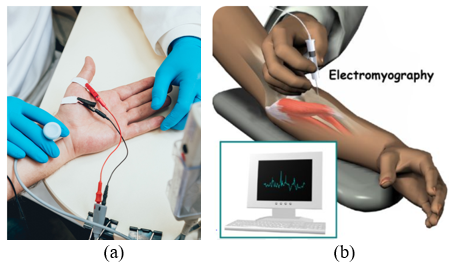Figure 1. (a) Non- invasive (b) Invasive techniques

EMG is used to detect the muscular information based on the contraction and expansion period of muscles through the electrodes, which are placed on the surface of the human body. The process of gathering information can be invasive or non- invasive. The process of conducting non-invasive surface EMG is performed using electrodes whereas the invasive EMG required a needle, which is being inserted into the patient body for collecting the muscle related information. Both the processes are shown in Figure 1.

The EMG model can be simply represented by Eq. (1).

$y(n)=\sum_{t=0}^{N-1} h(t) e(n-t)+x(n)$    (1)

y(n)$\rightarrow$ Modelled EMG signal.

e(n)$\rightarrow$ Fringing impulse.

h(t)$\rightarrow$ Motor Unit Action Potentials (MUAPs), which is used to provide significant source of information that is useful for diagnosis of neuromuscular disorders.

x(n)$\rightarrow$ While Gaussian Noise.

n $\rightarrow$ Number of motor unit.

This research deals with the Attribute selection and classification of the EMG signal into two categories that is either pain or normal activity of muscles.

2. Related Work

The research has been done by number of authors to enhance the Attribute selection and classification rate of the EMG signal by using different techniques. A survey has been conducted to know about the tradition techniques used by the previous authors and how one can improve the classification rate. Robotic learning was applied by Stiyal on EMG signals as a subject-independent framework . EMG classification can be utilized in distinct medical domains like as neuromuscular disorder diagnosis , Neuromuscular Disorder , knee pathology detection , motion recognition , fatigue muscle analysis , and prosthesis control .

Mishra et al. had classified EMG signals, which have been collected from bicep muscels or different category of patients such as normal, mayopathic and neuropathic. The time and frequency domain parameters of MUAP potential has been analysed and optimized using soft computing approach. The classification has been performed by RBFN, K-NN and SVM technique and has been observed that SVM performed well among all with an accuracy of 95.25% . de Dieu Uwisengeyimana and Ibrikci  have diagnose knee related problems using KNN and ANN classifiers. The data has been collected from the four muscles surrounding the knee and about 500 samples have been prepared. From the experiment it has been concluded that knee pathology can be better analyzed using ANN with detection accuracy of 91.3%. Lin et al.  have presented an attribute selection approach for EMG signal that helps to classify signal. Initially, data is pre-processed using normalization in order to minimize the effect of the inter- and intra-participant of signal resulting while collecting signal through sensors. Basically, three types of normalizations have been applied such as (i) channel wise, (ii) motion-wise and (iii) participant wise. Also, down sampling has been applied to remove the unwanted or overlapped data points. Using Base classifier such as ANN provides better accuracy of $83 \mp 6$ .

Pancholi and Joshi  presented EMG signal for upper limb. The data has been collected from five different positions of arms during the exercise period. palpation method has been used for the selection of muscles. Using palpation method, the nerves have been selected based on the blood flow. Using 29 subjects the signal has been acquired and the data has been divided into time domain and frequency domain features. For classification different classifiers such as Random Forest (RF), k-nearest neighbours (k-NN), linear discriminant analysis (LDA), Support Vector Machine (SVM), Random Tree (RT) have been used and the detection accuracy ranges from 57.69% to 99.92% .

Morbidoni et al.  have worked to deal with the classification of stance and swing as muscular disease using EMG classification approach. The classification of the designed system has been tested using Multi-Layer Perceptron techniques and the examined accuracy lies between 92.6%–97.2%. The study has suggested that ANN can be an appropriate tool for automatic classification of EMG signal .

3. Proposed Work

The entire work is shown in Figure 2, which consists of three main parts such as attribute row selection using genetic Algorithm (GA), Similarity measure using Cosine Similarity, application of critic method to normalize features and classification using Support Vector machine (SVM).

3.1 Dataset

The dataset is collected from https://www.kaggle.com/nccvector/electromyography-emg-dataset link. The considered dataset contains pain and normal muscular data of 1000 row for each category with seven different un-named attributes. The electric potential observed is as like presented in Table 1.

Table 1. Dataset

 Patient Number t1 t2 t3 t4 t5 t6 t7 t8 1 0.03125 0.054688 0.03125 0.039063 0.03125 0.046875 0.023438 0.023438 2 0.046875 0.03125 0.039063 0.039063 0.03125 0.023438 0.023438 0.015625 3 0.046875 0.023438 0.039063 0.054688 0.03125 0.023438 0.015625 0.023438 4 0.0625 0.039063 0.054688 0.039063 0.023438 0.03125 0.039063 0.015625 5 0.046875 0.03125 0.03125 0.03125 0.015625 0.03125 0.023438 0.03125 6 0.03125 0.054688 0.054688 0.078125 0.03125 0.023438 0.023438 0.03125 7 0.015625 0.046875 0.03125 0.03125 0.03125 0.023438 0.023438 0.03125 8 0.039063 0.03125 0.054688 0.054688 0.039063 0.023438 0.039063 0.023438 9 0.054688 0.054688 0.039063 0.039063 0.03125 0.023438 0.03125 0.03125 10 0.03125 0.03125 0.0625 0.054688 0.03125 0.015625 0.015625 0.015625 11 0.03125 0.039063 0.054688 0.039063 0.03125 0.039063 0.03125 0.03125 12 0.03125 0.023438 0.046875 0.0625 0.039063 0.023438 0.023438 0.023438 13 0.03125 0.046875 0.039063 0.0625 0.03125 0.023438 0.039063 0.023438 14 0.023438 0.023438 0.070313 0.078125 0.03125 0.023438 0.03125 0.023438 15 0.039063 0.070313 0.09375 0.054688 0.046875 0.023438 0.03125 0.03125 16 0.039063 0.023438 0.039063 0.046875 0.046875 0.03125 0.023438 0.023438 17 0.015625 0.046875 0.039063 0.054688 0.03125 0.015625 0.03125 0.039063 18 0.023438 0.039063 0.070313 0.085938 0.039063 0.03125 0.039063 0.015625 19 0.03125 0.039063 0.09375 0.0625 0.023438 0.03125 0.039063 0.023438 20 0.054688 0.070313 0.078125 0.078125 0.03125 0.015625 0.023438 0.015625 21 0.023438 0.046875 0.0625 0.0625 0.03125 0.023438 0.039063 0.023438

Where, t is the time interval for time ranging from t1 to t8 measured in miliseconds.

## 2.pngFigure 2. Flow of proposed work

3.2 Attribute selection

Attribute selection of EMG signal is performed on each recorded movement data per session using the electrodes placed on the human body. The amplitude variation in the EMG is high when any movement is occurs otherwise the signals are in rest and amplitude variation reduces. EMG signal, each voltage-time amplitude value need Attribute selection to find out the relevant features for painful data or normal data to achieve better classification accuracy. The benchmark for the selection and rejection is relative to the value which is being used. It means, the selection and rejection will depend upon the other relative values available in that class of other patients.

In this research, attribute selection of the uploaded EMG signal is performed using nature inspired Genetic Algorithm. GA selects rows among the available dataset as per the designed fitness function represented by Eq. (2).

Fitness function$=1 \quad(1-e) \times F s>F t$

0 Otherwise            (2)

where, Fs = Current Attributes,

Ft = All Attributes Row Values.

Each row has been tested using Eq. (2). If the row satisfied the fitness function, then categorize it either pain or normal. Otherwise, repeat the process for next row. Using this process, the unwanted signal has been removed and the row also obtained in reduced and desired form. The process of GA is shown in Figure 3.

## 3.png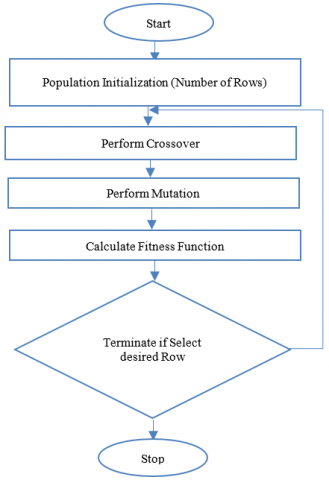Figure 3. GA process

Step 1: Initialization of Population (Rows): Initialize, population string (raw EMG data) known as chromosomes. To resolve problem that is to select EMG signal, which is being recorded during motion and to reject the EMG signal, which is recorded at rest position has been performed based on the designed fitness function.

Step 2: Selection: In this step, the selection of appropriate signal, which is higher than fitness value is eliminated and those less than or equal to fitness value are selected. Those values of rows that have lowest value are known as parent and contributed to the generation of new member named as children.

Step 3: Mutation: helps to search with the best row selection based on mutation threshold.

Step 4: Termination: The Attribute selection process is terminated while the desired rows are selected and categorized as pain and normal signal [17, 18].

Feature selection using GA

 Required Input: EMG Feature Data$\leftarrow$Extracted feature from used EMG Dataset for Pain & Normal Categories Fitness Function$\leftarrow$Designed fitness function for feature selection Fitness function = 1        (1-e) X Fs>Ft                               0        Otherwise Where, e = It is the generated mutation error Fs = Current Attributes Ft = All Attributes Row Values Obtained Output: OEMG-FD$\leftarrow$Optimized EMG Feature Data Start GA Load Dataset, EMGFeature Data (EMG-FD) = Load feature attributes To optimized the EMG-FD, GA is used Set GA Parameters: Population Size (P) – Based on the number of properties                                            CO – Crossover Operators                                            MO – Mutation Operators Calculate Length of EMG-FD in terms of Len Set,Optimized EMG Feature Data, OEMG-FD = [] For I = 1 $\rightarrow$ Len      $\mathrm{Fs}=\mathrm{EMG}-\mathrm{FD}(\mathrm{I})=$Selected$_{\text {EMG Attributes}}$     $\mathrm{Ft}=$ Threshold $_{\text {Attributes}}=\sum_{i=1}^{R} E M G-F D(I)$ $F(f)=$ Fit Fun $\left(e, F_{s}, F_{t}\right)$      Nvar = Number of variables      BestProp = OEMG-FD = GA (F(f), T, Nvar, GA Parameters) End - For Return: OEMG-FD as an Optimized EMG Feature Data End – Function

3.3 Cosine similarity

Cosine similarity is being applied on the selected rows, which return a single similarity index for each row. Suppose, the selected rows after GA of 700 has been obtained from the available 1000 rows for both pain and normal EMG signals. Therefore, similarity has been measured for each row with the remaining 699 rows. In this way a single or average value has been obtained using Eq. (3).

Avg $\operatorname{sim}=\frac{\sum_{i=1}^{n} \cos _{\operatorname{sim}}}{n}$            (3)

where, n is total number of similarities

After obtaining the average value, 20% of value has been added and subtracted to the obtained average similarity value as denoted by $b_{1}$ and $b_{2}$ . Mathematically, can be represented by Eq. (4);

$B_{1}=\operatorname{Avg} \operatorname{Sim}+\frac{A v g \operatorname{sim} N o.}{100}$

$B_{2}=\operatorname{Avg} \operatorname{sim}-\frac{\operatorname{Avg} \operatorname{Sim} \mathrm{No} .}{100}$            (4)

If the value of $B_{1}$ and $B_{2}$ lies between the similarity value and those values are used for the training and classification purpose using SVM approach. Otherwise drop the data .

Cosine similarity

 Required Input: OEMG-FD $\leftarrow$ Optimized EMG Feature Data Obtained Output: SimCos$\leftarrow$ Cosine similarity between OEMG-FD Avg Sim $\leftarrow$ Average Similarity Start Create an empty array to store similarity, SimCos = [] Sim-count = 0 For I = 1 $\rightarrow$ Length (OEMG-FD)       Current_Data = OEMG-FD (I)       For J = I+1 $\rightarrow$ Length (OEMG-FD)             L = |Cos (Current_Data) - Cos (Data (J))|             SimCos [sim_count, 1] = Current_Data             SimCos [sim_count, 2]= Data(J)             SimCos [sim_count, 3]=L              Increment in array, Sim-count = Sim-count+1       End-For End – For Calculate Average Similarity Avg $\operatorname{sim}=\frac{\sum_{i=1}^{n} \operatorname{sim} \cos }{n}$// Where, n is total number of similarities Return: SimCos as an output in terms of cosine similarity between OEMG-FD and Avg Sim as an Average Similarity End-Function

3.4 Critical method

Critic method is used to normalize the feature vector attained from the application of Genetic Algorithm . Critic method acts on three elements as follows:

1. The current state of value

2. The maximum value of the section

3. The minimum value of the section

Following pseudo code is applied in order to implement the critic method.

Application of Critic

 For $_{\text {each}}$ Selected row Foreach col in Selected row Cr $_{\text {Feature}_{\text {Value}}}=$Selected$_{\text {row-col}}$. Feature Threshold. Value $=\frac{\sum_{i=1}^{n} \text { Feature }_{\text {Value}}}{n}$ If Creature_{Value} $\geq$Thre shold$_{\text {Value}}$ Do $_{\text {Nothing}}$ Else Find maxv=Maximum Value(col) of all rows Find $\min _{v}=$ Minimum Value $($col$)$ of all rows $R v=\frac{C r_{\text {Featurevalue}}-\min _{v}}{\max _{v}+\min _{v}}$ Replace $\mathrm{Cr}_{\text {Feature}_{\text {value}}}$ by $\boldsymbol{R} \boldsymbol{v}$ End For End For

The critic method takes the current attribute value and checks it with the average value of other members which is called the selection value in this case. If the attribute value is less than the threshold value, it checks for the maximum and minimum value of this attribute in all the available rows of the category. The current attribute value is subtracted from the min value and is divided by a total of max and min value. The outcome is replaced by the current attribute value.

3.5 Support Vector Machine (SVM)

SVM is a machine learning approach used to classify EMG signal as pain and normal. Using this approach, a hyperplane has been constructed to distinguished two different data as normal and pain in this case. To train SVM, let the input train data be as: $\left(a_{1} b_{1}\right),\left(a_{2} b_{2}\right), \ldots,\left(a_{m} b_{m}\right) \epsilon P^{N} \times\{-1,+1\}$.

$a_{i} \rightarrow$ input value,

$b_{i} \rightarrow$ Assigned class to which input belongs {-1,+1}.

In case, if the input data is not separated linearly, then a transform of ($\varphi: P^{N} \rightarrow P^{M}$) has been used with a new feature space represented by $P^{M}$.

Using this as a function, the obtained hyperplane can be separated as per the Eq. (5);

$\omega \times \varphi(a)+b=0$

$\omega \in P^{M}$ and $b \in P$           (5)

The training can be said best with optimal hyperplane and a minimum error. In case, if the signals are too close or overlap with each other, then a kernel function is used to separate that data. The kernel function might be Radial Basic Function (RBF), polynomial, linear, a Gaussian etc. [1, 22].

The training and testing using SVM is shown in Figure 4.

Classification using SVM

 Required Input: OEMG-FD $\leftarrow$Training Data as an Optimized EMG Feature Data C $\leftarrow$Target/Category in terms of Pain and Normal RBF $\leftarrow$ Radial Basis Function as a Kernel Function SimCos $\leftarrow$ Cosine similarity between OEMG-FD Avg Sim $\leftarrow$ Average Similarity Obtained Output: SVM-Structure $\leftarrow$ Trained SVM Structure Start Calculate \begin{aligned} B_{1} &=\operatorname{Avg} \operatorname{sim}+\frac{A v g \operatorname{Sim} N o.}{100} \\ B_{2} &=\operatorname{Avg} \operatorname{sim}-\frac{\operatorname{Avg} \operatorname{Sim} N o .}{100} \end{aligned} If Sim Value < B1& Sim Value > B2 Initialize the SVM with training data OEMG-FD with RBF as Kernel function For I = 1 $\rightarrow$ Length (OEMG-FD)              If OEMG-FD (I) == Pain                   Cat (1) = OEMG-FD (I)              Else                   Cat (2) = OEMG-FD (I) End – If        End – For Else       Drop OEMG-FD End – If VM-Structure =SVMTRAIN (OEMG-FD, Cat, Kernel function) Return: SVM-Structure as a Trained SVM structure End-Function

## 4a.png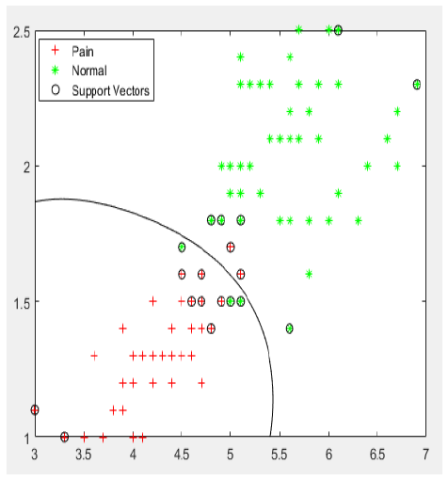## 4b.png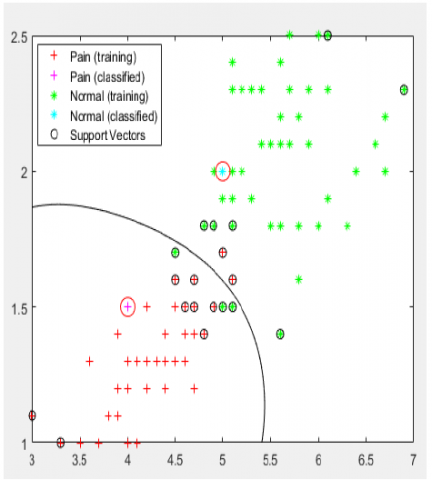Figure 4. Training and testing of EMG Signal using SVM

4. Result and Discussions

The results of the designed Attribute selection and classification EMG system has been performed in MATLAB simulator. The aim of this research is to distinguish the pain and normal muscles using GA as an Attribute selection approach. To enhance the training of SVM, cosine similarity has been applied which will further reduce the irregular and the noise signal present in the available segmented EMG data. As the signal is filtered by two techniques such as GA and cosine similarity. Therefore, the possibility of detection accuracy also increases. The analysed values in terms of precision, recall, F-measure and classification accuracy are performed using Eq. (6), Eq. (7), Eq. (8) and Eq. (9) respectively.

Precision$=\frac{T_{p}}{T_{p}+F_{p}}$        (6)

Recall$=\frac{T_{p}}{T_{p}+F_{n}}$           (7)

$F-$Measure$=\frac{2 \times \text {Precision } \times \text { Recall}}{\text {Precision }+\text { Recall}}$         (8)

Accuracy$=\frac{T_{p}+T_{N}}{T_{p}+F_{p}+F_{n}+T_{N}}$        (9)

Here, $T_{p} \rightarrow$ The EMG signal that are actually comes under pain or normal category and also predicted as the same.

$F_{n} \rightarrow$ the EMG signal that are being predicted as real but are noise or unwanted signal considered at rest position.

$F_{p} \rightarrow$ The EMG signal that is actually real but predicted as undesired or noisy signal.

$T_{n} \rightarrow$ The number of appropriately predicted real signal.

Precision values analysed for GA with SVM, GA +Cosine Similarity+ SVM for normal and pain muscular signal is shown in Figure 5 with the values listed in Table 2.

From Figure 5, it is clearly seen that maximum values of precision are analysed for the proposed work i.e. (GA+ Cosine Similarity +SVM) approach used for normal EMG signal followed by GA+ Cosine Similarity +SVM used for classifying pain EMG signal. The average precision values examined for the proposed work using GA SVM and GA with Cosine similarity with SVM for normal EMG signal are 0.8456 and 0.9804 respectively. Similarly, the precision values analyzed for the pain EMG signal using GA with SVM and GA with Cosine similarity with SVM are 0.815 and 0.943 respectively. We observed that, precision rate is improved by using the combination of cosine similarity measurement technique along with the GA and SVM. Improved precision rate indicates the selection of EMG attributes are better during the classification process and rate of true features is high due to better training of system.

The recall values for the pain and normal muscles analyzed using automatic classification system are summarized in Table 3 and graphically compared in Figure 6. The average values of recall measured for normal EMG signal using GA with SVM, and GA+ Cosine Similarity +SVM are 0.7456 and 0.7501. Similarly, the average value of recall examined for pain muscular signal using GA with SVM, and GA+ Cosine Similarity +SVM are 0.7451 and 0.838 respectively. Similar to precision, recall rate also improved by using the combination of cosine similarity measurement technique along with the GA and SVM. Recall rate denotes the selection of appropriate feature according to the training of the system and in proposed work the recall rate is improved that means proposed system achieved good performance.

Table 2. Computed precesion

 Number of Iterations GA with SVM GA+ Cosine Similarity +SVM Normal Pain Normal Pain 1 0.853 0.835 0.992 0.985 2 0.847 0.829 0.972 0.942 3 0.839 0.801 0.983 0.957 4 0.835 0.799 0.972 0.936 5 0.825 0.812 0.995 0.943 6 0.817 0.794 0.978 0.928 7 0.815 0.752 0.968 0.933 8 0.857 0.824 0.986 0.947 9 0.895 0.852 0.976 0.927 10 0.873 0.853 0.982 0.937

## 5.png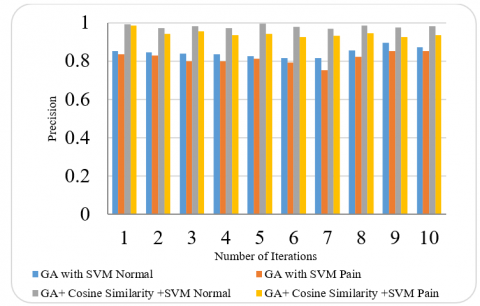Figure 5. Precision analysis

Table 3. Computed recall

 Number of Iterations GA with SVM GA+ Cosine Similarity +SVM Normal Pain Normal Pain 1 0.795 0.775 0.751 0.865 2 0.758 0.895 0.768 0.758 3 0.776 0.765 0.728 0.789 4 0.767 0.687 0.715 0.862 5 0.759 0.694 0.756 0.831 6 0.742 0.687 0.785 0.864 7 0.768 0.668 0.735 0.863 8 0.712 0.697 0.759 0.857 9 0.696 0.785 0.736 0.869 10 0.683 0.798 0.768 0.823

## 6.png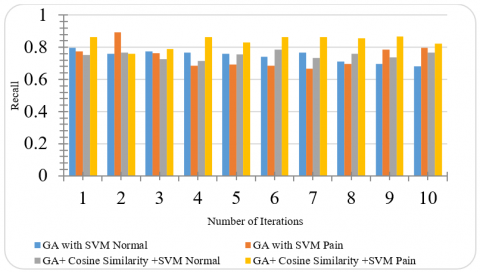Figure 6. Recall analysis

The analyzed data for F score is shown in Figure 7 with the values listed in Table 4 is the collective representation of precision and recall. The average value of F score analyzed for two different EMG data (pain and normal) using GA with SVM and GA with Cosine and SVM are represented by the orange, the yellow, the blue and the grey bar respectively. Here, F-score basically denotes the average of precision and recall rate and for a better system it should be high. From the observation we, concluded that, precision as well recall rate is better by using the combination of cosine similarity measurement technique.

The classification accuracy examined by the proposed work for pain and normal EMG signal are listed in Table 5 and graphically illustrated in Figure 8. From the figure it is clearly seen that the average accuracy for the painful EMG signal is higher than 70%. The pain and normal EMG signal has been classified with an average accuracy of 92.4% and 91.3% respectively.

Table 4. Computed F-score

 Number of Iterations GA with SVM GA+ Cosine Similarity +SVM Normal Pain Normal Pain 1 0.822979 0.803882 0.854839 0.921108 2 0.800032 0.860737 0.858041 0.840042 3 0.806271 0.782586 0.836498 0.864918 4 0.799557 0.738779 0.823924 0.897477 5 0.790625 0.748377 0.859189 0.883464 6 0.777696 0.736635 0.870936 0.894857 7 0.790802 0.707515 0.835561 0.896636 8 0.7778 0.755198 0.857735 0.899755 9 0.783055 0.817129 0.839178 0.897063 10 0.7664 0.824584 0.861915 0.876308

## 7.png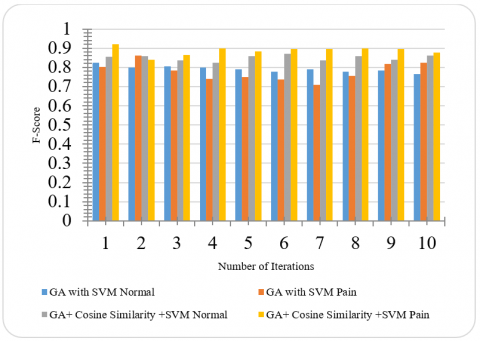Figure 7. F-score analysis

Table 5. Computed classification accuracy

 Number of Iterations GA with SVM GA+ Cosine Similarity +SVM Normal Pain Normal Pain 1 0.876 0.786 0.925 0.941 2 0.875 0.795 0.914 0.935 3 0.882 0.812 0.935 0.925 4 0.891 0.802 0.927 0.934 5 0.875 0.796 0.915 0.915 6 0.868 0.765 0.905 0.905 7 0.878 0.754 0.896 0.934 8 0.868 0.763 0.887 0.936 9 0.838 0.824 0.914 0.902 10 0.875 0.798 0.916 0.917

## 8.png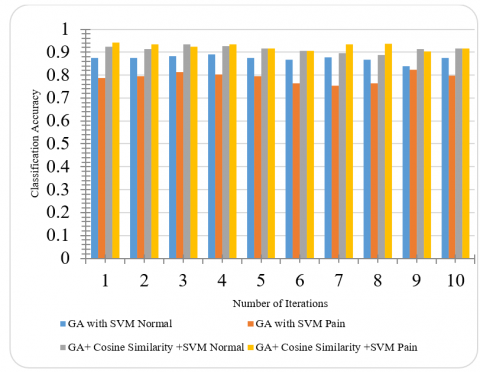Figure 8. Classification accuracy analysis

## 9.pngFigure 9. Accuracy comparison with the existing work

Table 6. Comparison of computed accuracy with the existing work

 Proposed Work Existing Work  Normal Pain Normal Pain 91.3 92.4 82.14 87.57

To show the effectiveness of the proposed work, the comparison of examined classification accuracy is shown in Table 6 and plotted for comparative analysis in Figure 9. The graph shows that the rate of classifying EMG signal whether it is normal EMG or pain EMG signal the proposed algorithm performed well compared to the existing ANN classifier. Also, the percentage increase in the classification rate of proposed work for normal EMG from the Jiang et al. 2019 work  is 11.15% whereas, for painful signal, the classification accuracy has been increased by 5.52%. This enhancement has been obtained because of the proper selection of appropriate EMG data which in turns increase the training rate and hence the classification during the testing process.

5. Conclusion

An automatic Attribute selection and classification system for EMG signal has been designed using GA with SVM as Attribute selection and classification techniques respectively.  The results show that proposed model worked well with higher classification rate for both pain and normal EMG signal. An appropriate selection of EMG signal has been performed using GA with cosine similarity as well as reduced the available data that minimized the training error and hence improve classification rate. The research can provide a better understanding of the EMG signal Attribute selection and classification procedure. The classification accuracy observed for normal and painful EMG signal are 91.3% and 92.4% respectively. Also, the improvement of the proposed work against the existing work of about 11.15% and 5.52% has been examined for normal and painful EMG signal against the existing work. In future, we plan to use artificial neural network as a classification approach or comparing the results of SVM and ANN in order to know the efficiency of the classifiers in terms of classification accuracy.

References

 Kaur, G., Arora, A.S., Jain, V. (2009). Multi-class support vector machine classifier in EMG diagnosis. WSEAS Transactions on Signal Processing, 5(12): 379-389.

 Chan, F.H., Yang, Y.S., Lam, F.K., Zhang, Y.T., Parker, P.A. (2000). Fuzzy EMG classification for prosthesis control. IEEE Transactions on Rehabilitation Engineering, 8(3): 305-311. https://doi.org/10.1109/86.867872

 Campos, D.P., Abatti, P.J., Bertotti, F.L., Gomes, O.A., Baioco, G.L., Hill, J.A.G., da Silveira, A.L.F. (2019). Ingestive pattern recognition on cattle using EMG segmentation and feature extraction. In: Costa-Felix, R., Machado, J., Alvarenga, A. (eds) XXVI Brazilian Congress on Biomedical Engineering. IFMBE Proceedings, pp. 281-288. https://doi.org/10.1007/978-981-13-2517-5_43

 Phinyomark, A., Campbell, E., Scheme, E. (2020). Surface electromyography (EMG) signal processing, classification, and practical considerations. In: Naik G. (eds) Biomedical Signal Processing. Series in BioEngineering. Springer, Singapore. https://doi.org/10.1007/978-981-13-9097-5_1

 Stival, F. (2018). Subject-independent frameworks for robotic devices: Applying robot learning to EMG signals. Padova Digital University Archive.

 Bhuvaneswari, P., Kumar, J.S. (2016). Electromyography based detection of neuropathy disorder using reduced cepstral feature. Indian Journal of Science and Technology, 9(8): 1-4. https://doi.org/10.17485/ijst/2016/v9i8/87899

 Benazzouz, A., Guilal, R., Amirouche, F., Slimane, Z.E.H. (2019). EMG feature selection for diagnosis of neuromuscular disorders. 2019 International Conference on Networking and Advanced Systems (ICNAS), Annaba, Algeria, pp. 1-5. https://doi.org/10.1109/ICNAS.2019.8807862

 Naik, G.R., Selvan, S.E., Arjunan, S.P., Acharyya, A., Kumar, D.K., Ramanujam, A., Nguyen, H.T. (2018). An ICA-EBM-based sEMG classifier for recognizing lower limb movements in individuals with and without knee pathology. IEEE Transactions on Neural Systems and Rehabilitation Engineering, 26(3): 675-686. https://doi.org/10.1109/TNSRE.2018.2796070

 Huang, Y., Liu, H. (2016). Performances of surface EMG and ultrasound signals in recognizing finger motion. 2016 9th International Conference on Human System Interactions (HSI), Portsmouth, pp. 117-122. https://doi.org/10.1109/HSI.2016.7529618

 Zoppirolli, C., Pellegrini, B., Bortolan, L., Schena, F. (2016). Effects of short-term fatigue on biomechanical and physiological aspects of double poling in high-level cross-country skiers. Human Movement Science, 47: 88-97. https://doi.org/10.1016/j.humov.2016.02.003

 Pancholi, S., Joshi, A.M. (2018). Portable EMG data acquisition module for upper limb prosthesis application. IEEE Sensors Journal, 18(8): 3436-3443. https://doi.org/10.1109/JSEN.2018.2809458

 Mishra, B., Wadhwani, A.K., Singh, S. (2019). EMG signal classification for neuromuscular disorder using soft-computing techniques. IJIRMPS-International Journal of Innovative Research in Engineering & Multidisciplinary Physical Sciences, 7(1).

 de Dieu Uwisengeyimana, J., Ibrikci, T. (2017). Diagnosing knee osteoarthritis using artificial neural networks and deep learning. Biomedical Statistics and Informatics, 2(3): 95-102. https://doi.org/10.11648/j.bsi.20170203.11

 Lin, J.F.S., Samadani, A.A., Kulić, D. (2016). Segmentation by data point classification applied to forearm surface EMG. In: Leon-Garcia A. et al. (eds) Smart City 360°. SmartCity 360 2016, SmartCity 360 2015. Lecture Notes of the Institute for Computer Sciences, Social Informatics and Telecommunications Engineering, vol 166. Springer, Cham. https://doi.org/10.1007/978-3-319-33681-7_13

 Pancholi, S., Joshi, A.M. (2018). Portable EMG data acquisition module for upper limb prosthesis application. IEEE Sensors Journal, 18(8): 3436-3443. https://doi.org/10.1109/JSEN.2018.2809458

 Morbidoni, C., Principi, L., Mascia, G., Strazza, A., Verdini, F., Cucchiarelli, A., Di Nardo, F. (2019). Gait phase classification from surface EMG signals using Neural Networks. In: Henriques J., Neves N., de Carvalho P. (eds) XV Mediterranean Conference on Medical and Biological Engineering and Computing – MEDICON 2019. MEDICON 2019. IFMBE Proceedings, vol 76. Springer, Cham. https://doi.org/10.1007/978-3-030-31635-8_9

 Ambikapathy, B., Kirshnamurthy, K., Venkatesan, R. (2018). Assessment of electromyograms using genetic algorithm and artificial neural networks. Evolutionary Intelligence, 1-11. https://doi.org/10.1007/s12065-018-0174-0

 Karimi, M., Pourghassem, H., Shahgholian, G. (2011). A novel prosthetic hand control approach based on genetic algorithm and wavelet transform features. 2011 IEEE 7th International Colloquium on Signal Processing and its Applications, Penang, pp. 287-292. https://doi.org/10.1109/CSPA.2011.5759889

 Hunter, P. (2016). Margin of error and confidence levels made simple.

 Sidorov, G., Gelbukh, A., Gómez-Adorno, H., Pinto, D. (2014). Soft similarity and soft cosine measure: Similarity of features in vector space model. Computación y Sistemas, 18(3): 491-504.

 Alinezhad, A., Khalili, J. (2019). CRITIC method. In: New Methods and Applications in Multiple Attribute Decision Making (MADM). International Series in Operations Research & Management Science, vol 277. Springer, Cham. https://doi.org/10.1007/978-3-030-15009-9_26

 Alkan, A., Günay, M. (2012). Identification of EMG signals using discriminant analysis and SVM classifier. Expert systems with Applications, 39(1): 44-47. https://doi.org/10.1016/j.eswa.2011.06.043

 Jiang, M., Mieronkoski, R., Syrjälä, E., Anzanpour, A., Terävä, V., Rahmani, A.M., Salanterä, S., Aantaa, R., Hagelberg, N., Liljeberg, P. (2019). Acute pain intensity monitoring with the classification of multiple physiological parameters. Journal of Clinical Monitoring and Computing, 33(3): 493-507. https://doi.org/10.1007/s10877-018-0174-8